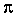SEARCH HOMEMath Central Quandaries & QueriesQuestion from ed: an air duct in the form of a circular cylinder has a cross section if diameter 16 in. the distance between the bases is 20 ft and the elements are inclined at an angle of 50 degrees to the bases. find the amount of magnesia required to protect the duct with magnesia covering 1/2 in thick? tnxHi Ed.

First, let's consider those angled ends. You might assume they have an effect on the amount of coating, but they don't! Remember that you can mentally attach two such cylinders together end-to-end and you don't have any waste - your cuts are still every 20 feet, just angled, and you'd have the same amount of internal surface to cover to the same depth for any angle - 90 degrees, 50 degrees or anything else.

It is interesting that the coating is so thick! If the coating were very thin, you'd just apply it to the duct material before rolling it up into a cylinder. Then you'd just think of it as length x width x depth (length: 20 ft, width (the circumference of the duct): 16inches; depth 0.5 inches). But you know that if you have something very thick, you cannot simply roll a flat slab of material into a tube without affecting the thickness - the outside surface is stretched and the inside surface is compressed. When the thickness of the coating is very much less than the radius of the tube, this is a negligible factor, but I think 1/2 inch is quite significant, so instead of thinking of the coating as kind of box-shaped (length, width and depth at right angles), let's actually think of it as tube shaped!

The outside radius of the coating (the coating is applied to the inside of the duct) is the same as the duct's inside radius: 8 inches. The inside radius of the coating is 1/2 inch less. Therefore, the "ring" (mathematical term: annulus) has the area we are interested in finding, because that is filled with the coating material. The area of a ring is simply the area of the outside circle minus the area missing: the inside circle. So that means 82minus (7.5)2square inches. My calculator gives 24.35 square inches. When I multiply this by 20 feet, that makes 24.35 x 12 x 20 = 5843 cubic inches of coating material.

Just for interest, let's compare that to the result we'd have with the "coating as a box shape" method: (20 x 12) x 16x 0.5 = 6032 cubic inches. That's more than 3% of a difference, which is maybe not significant for one duct, but for a ducting company it would be a serious consideration.

Cheers,
Stephen La RocqueMath Central is supported by the University of Regina and The Pacific Institute for the Mathematical Sciences.